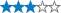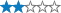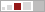##### This cache has been archived.

parmstro: Gradually archiving most of our caches as we have other priorities in life now.

More
<

## Drago Dormiens Nunquam Titillandus

A cache by Professor Dumbledore Message this owner
Hidden : 06/28/2007
Difficulty:Terrain:Size:(regular)

#### Watch

How Geocaching WorksPlease note Use of geocaching.com services is subject to the terms and conditions in our disclaimer.

### Geocache Description:

This started as a cooperative cache. Now the answers are all there and it is a straight puzzle cache.

I have asked a series of questions based on each book, which led to some numbers.

The final calculations will require some extra Harry Potter knowledge, which can be answered without the books.

There is also a very apt FTF from mugglenet.com.

Quick bonus question - what does the school motto mean?

Answered correctly by ALBOY - "Never tickle a sleeping dragon"

Part 1 - a gentle start to get you going:

Q1.How many Knuts in a Galleon? Answer: 29x17 = 493; page 58.

Q2.Which birthday did Nicolas Flamel celebrate last year? (as quoted in book, don't recalculate for today) Answer: 665; page 161.

Q3.What date in July was the break-in at Gringotts? Answer: 31; page 105. By the way - that's Harry's birthday.

Q4.Number of balls in Quidditch times number of players on each team. Answer: 4x7 = 28; balls page 61, players page 81.

Q5.(Length of Voldermort's wand minus length of Harry's) times Dursley's house number. Answer: (13.5 - 11) = 2.5 x 4 = 10; page 65 and page 7.

Q6.How many bottles on the table? Answer: 7; page 206.

Q7.What type of Nimbus does Harry get? Answer: 2000; page 122.

Calculations:

Add all the page numbers for part 1 together (e.g. for Q4 you would include 61 and 81) = A, and add all the other numbers in all the answers for part 1 together (e.g. for Q5 you would include 13.5, 11, 2.5, 4 and 10) = B

Part 2 - entering the Chamber

Q1.How many times does Ron buff up the Quidditch Cup? Answer: 14; page 93.

Q2.How many eyes does Aragog have? Answer: 8; page 205.

Q3.How many times has Gilderoy won the Witch Weekly's Most-Charming-Smile Award? Answer: 5; page 71.

Q5.How many muggles have been claimed by the necklace? Answer: 19; page 44.

Q6.How many school governors? Answer: 12; page 194.

Q7.How many days do lacewings have to be stewed for in the Polyjuice recipe? Answer: 21; page 125.

Calculations:

Add all the page numbers for part 2 together = C, and add all the other numbers in all the answers for part 2 together = D

Part 3 - get out of prison

Q2.How much extra for 'ot chocolate? Answer: 2; page 31.

Q3.How many registered : Animagi this century? Answer: 7; page 257.

Q4.How many secret passages does Filch know about? Answer: 4; page 144.

Q5.By how many points does Gryffindor lose to Hufflepuff in Quidditch? Answer: 100; page 135.

Q6.How many fake Dementors appear at the match? Answer: 3; page 193.

Q7.How many points did Neville get for fighting the boggart? Answer: 10; page 105.

Calculations:

Add all the page numbers for part 3 together = E, and add all the other numbers in all the answers for part 3 together = F

Part 4 - how many will be selected?

Q1.How many points did Harry get for the first task? Answer: 8+9+9+10+4=40; pages 314 and 315.

Q2.And for the second? Answer: 45; page 440.

Q3.How many "objects forbidden inside the castle this year"? Answer: 437; page 162.

Q4.How many times does Dean hop round the room? Answer: 3; page 203.

Q5.How many points did Ireland get? Answer: 170; page 103.

Q6.How many people does Karkaroff name? Answer: 6; pages 511, 512 and 513.

Q7.How many people appear out of Voldermort's wand? Answer: 5; pages 577, 578 and 579.

Calculations: think you can guess!

Add all the page numbers for part 4 together = G, and add all the other numbers in all the answers for part 4 together = H

Part 5 - Dumbledore's Army

Q1.How many floors are there in St Mungo's? Answer: 6; page 429

Q2.How many doors are there in the circular room? Answer: 12; page 680.

Q3.How many OWLs each did Fred and George get? Answer: 3; page 205.

Q4.Take the level number for Magical Law Enforcement away from the level number for Magical Games and Sports. Answer: 7-2=5; pages 119 and 120.

Q6.What decree number bans The Quibbler? Answer: 27; page 512.

Q7.What number Diagon Alley is Fred and George's shop? Answer: 93; page 595.

Calculations: think you can guess!

Add all the page numbers for part 5 together = I, and add all the other numbers in all the answers for part 5 together = J

Part 6 - is Snape good or bad?

Q1.How old is Stan Shunpike when he is arrested? Answer: 21; page 208.

Q2.What time does Harry arrange to meet Luna for Slughorn's party? Answer: 8; page 291.

Q3.How many OWLs does Ron get? Answer: 7; page 101.

Q4,How many potions has Slughorn prepared? Answer: 4; pages 175 and 177.

Q6.At what time did Dumbledore arrange to pick up Harry from the Dursleys? Answer: 11; page 46.

Q7.How many points did Gryffindor win by in the final Quidditch match? Answer: 450-140=310; page 499.

Calculations: think you can guess!

Add all the page numbers for part 6 together = K, and add all the other numbers in all the answers for part 6 together = L

Part 7 - who wins?

Q1.How many Galleons for a Mudblood? Answer: 5; page 368.

Q2.What level is the Atrium? Answer: 8; page 218.

Q3.How many Principal Exceptions to Gamp's Law of Elemental Transfigur-? Answer: 5; page 241.

Q4.How many ghosts accompany Harry into the Forbidden Forest? Answer: 4; page 561.

Q5.How many bedrooms in Shell Cottage? Answer: 3; page 408.

Q6.How old was James Potter when he died? Answer: 21; page 268.

Q7.How old is Auntie Muriel? Answer: 107; page 118.

Calculations: think you can guess!

Add all the page numbers for part 7 together = M, and add all the other numbers in all the answers for part 7 together = N

The final calculations for the coords:

Assume 51 and 0 as usual

1.Calculate the average for pages and numbers:

Pages = (A+C+E+G+I+K+M-10)/7

Numbers = (B+D+F+H+J+L+N-1)/7

2.For West:

(Pages*7) + Numbers + 2*(number of staircases at Hogwarts)

3.For North:

West + Sirius' prison number + number of fouls at Quidditch + (Harry's day of birth * Harry's month of birth)

4.Take 1 away from each

5.This takes you to the post at Diagon Alley (nice walk from the Leaky Cauldron or the Three Broomsticks)

6.From here take the Northish path and walk one adult step for each breed of pure-bred dragon there is in the world

I have double checked these, but shout if you have any problems!!Fubhyq nyy or rnfl gb svaq ba Tbbtyr
Gnxr n puvyq be n avssyre

Decryption Key

A|B|C|D|E|F|G|H|I|J|K|L|M
-------------------------
N|O|P|Q|R|S|T|U|V|W|X|Y|Z

(letter above equals below, and vice versa)